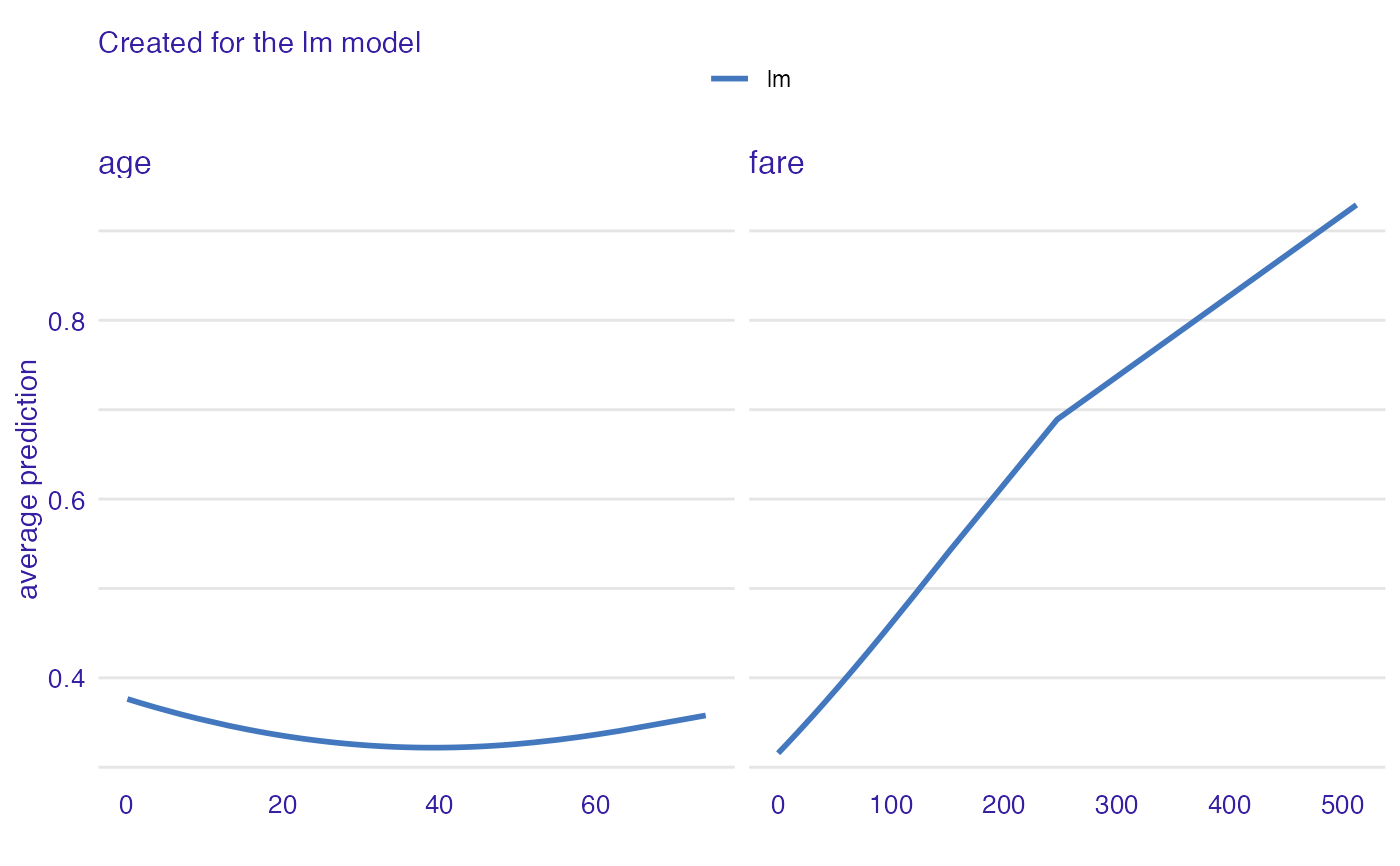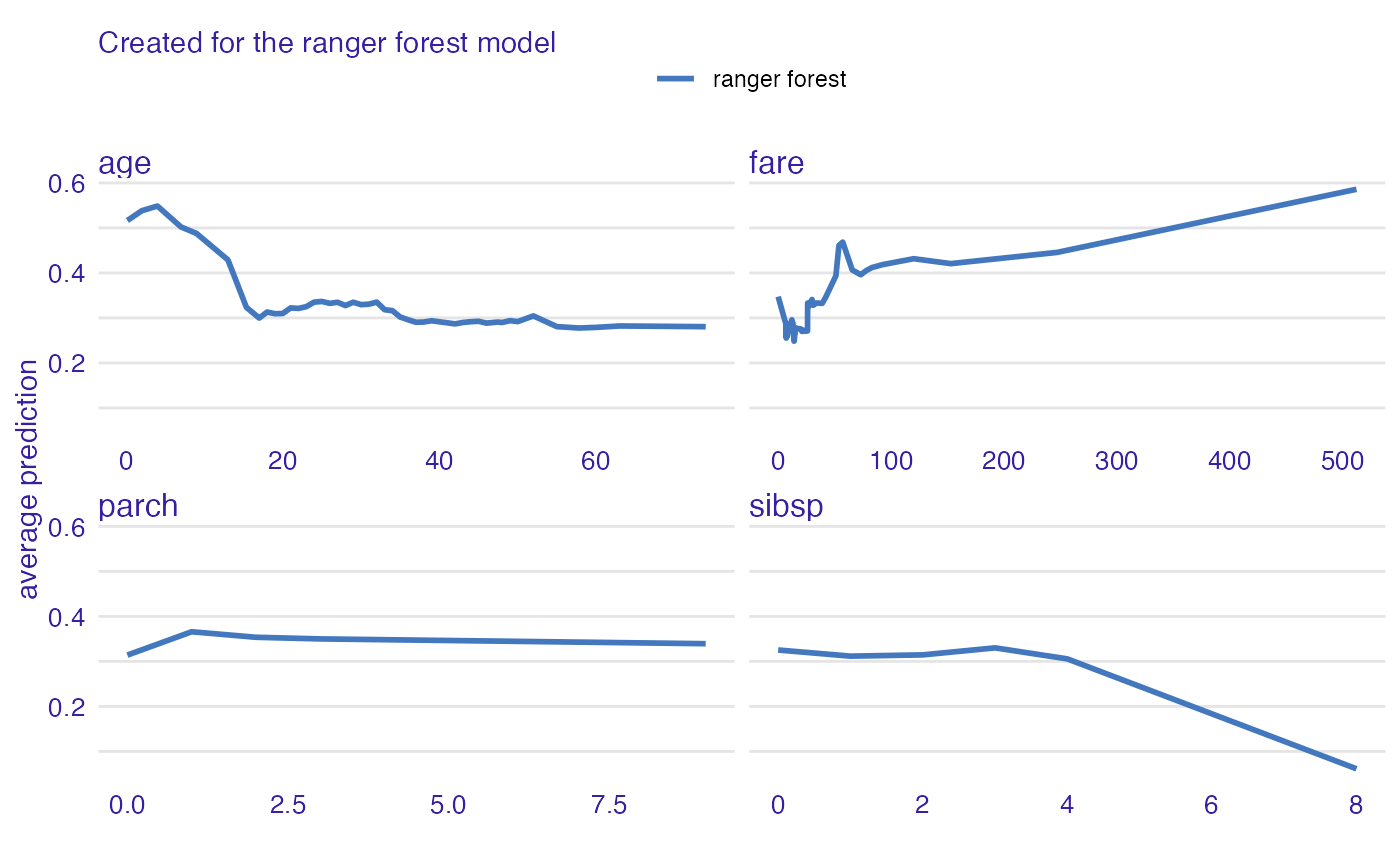Conditional Dependence Profiles (aka Local Profiles) average localy Ceteris Paribus Profiles. Function 'conditional_dependence' calls 'ceteris_paribus' and then 'aggregate_profiles'.

conditional_dependence(x, ...)

# S3 method for explainer
conditional_dependence(
x,
variables = NULL,
N = 500,
variable_splits = NULL,
grid_points = 101,
...,
variable_type = "numerical"
)

# S3 method for default
conditional_dependence(
x,
data,
predict_function = predict,
label = class(x),
variables = NULL,
N = 500,
variable_splits = NULL,
grid_points = 101,
...,
variable_type = "numerical"
)

# S3 method for ceteris_paribus_explainer
conditional_dependence(x, ..., variables = NULL)

local_dependency(x, ...)

conditional_dependency(x, ...)

## Arguments

x an explainer created with function DALEX::explain(), an object of the class ceteris_paribus_explainer or a model to be explained. other parameters names of variables for which profiles shall be calculated. Will be passed to calculate_variable_split. If NULL then all variables from the validation data will be used. number of observations used for calculation of partial dependence profiles. By default 500. named list of splits for variables, in most cases created with calculate_variable_split. If NULL then it will be calculated based on validation data avaliable in the explainer. number of points for profile. Will be passed to calculate_variable_split. a character. If "numerical" then only numerical variables will be calculated. If "categorical" then only categorical variables will be calculated. validation dataset, will be extracted from x if it's an explainer NOTE: It is best when target variable is not present in the data predict function, will be extracted from x if it's an explainer name of the model. By default it's extracted from the class attribute of the model

## Value

an object of the class aggregated_profile_explainer

## Details

Find more details in the Accumulated Local Dependence Chapter.

Explanatory Model Analysis. Explore, Explain, and Examine Predictive Models. https://ema.drwhy.ai/

## Examples

library("DALEX")
library("ingredients")

model_titanic_glm <- glm(survived ~ gender + age + fare,
data = titanic_imputed, family = "binomial")

explain_titanic_glm <- explain(model_titanic_glm,
data = titanic_imputed[,-8],
y = titanic_imputed[,8],
verbose = FALSE)

cdp_glm <- conditional_dependence(explain_titanic_glm,
N = 150, variables = c("age", "fare"))
#> Top profiles    :
#>                     _vname_ _label_        _x_    _yhat_ _ids_
#> age.lm.0.1666666667     age      lm  0.1666667 0.3762917     0
#> age.lm.2                age      lm  2.0000000 0.3713934     0
#> age.lm.4                age      lm  4.0000000 0.3662976     0
#> age.lm.7                age      lm  7.0000000 0.3591476     0
#> age.lm.9                age      lm  9.0000000 0.3547151     0
#> age.lm.13               age      lm 13.0000000 0.3466645     0plot(cdp_glm)# \donttest{
library("ranger")

model_titanic_rf <- ranger(survived ~., data = titanic_imputed, probability = TRUE)

explain_titanic_rf <- explain(model_titanic_rf,
data = titanic_imputed[,-8],
y = titanic_imputed[,8],
label = "ranger forest",
verbose = FALSE)

cdp_rf <- conditional_dependence(explain_titanic_rf, N = 200, variable_type = "numerical")
plot(cdp_rf)cdp_rf <- conditional_dependence(explain_titanic_rf, N = 200, variable_type = "categorical")
plotD3(cdp_rf, label_margin = 100, scale_plot = TRUE)
# }